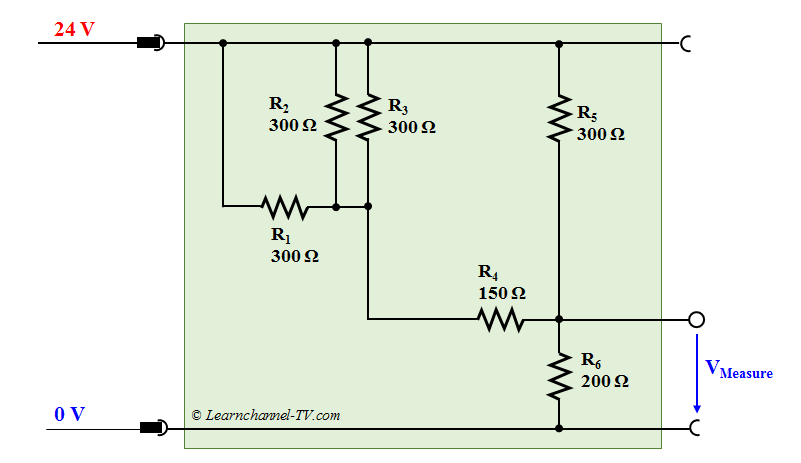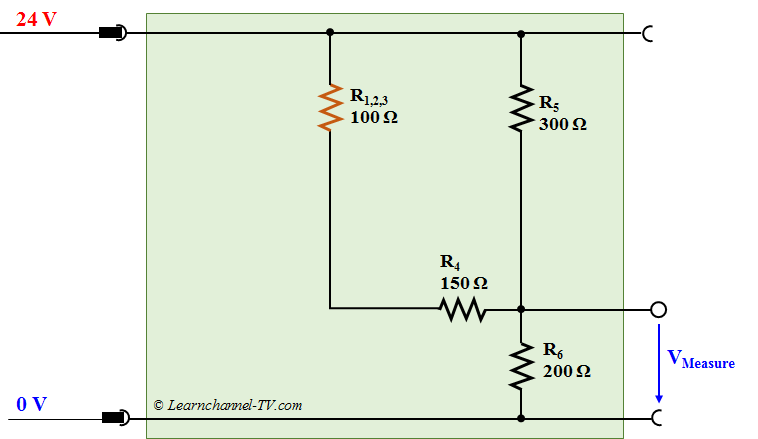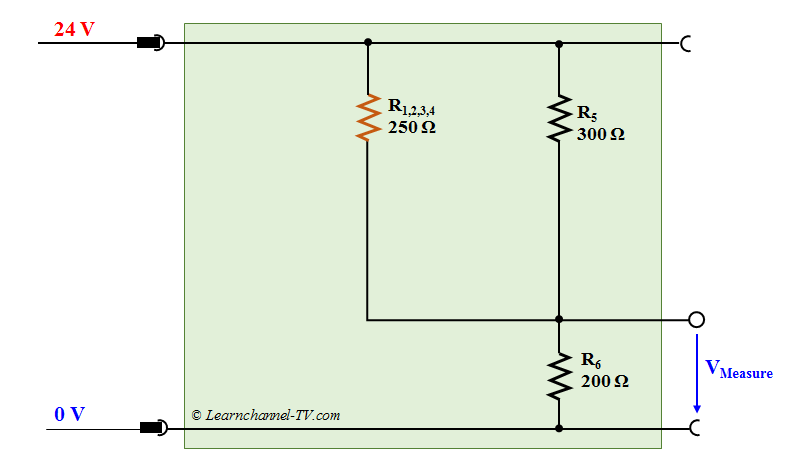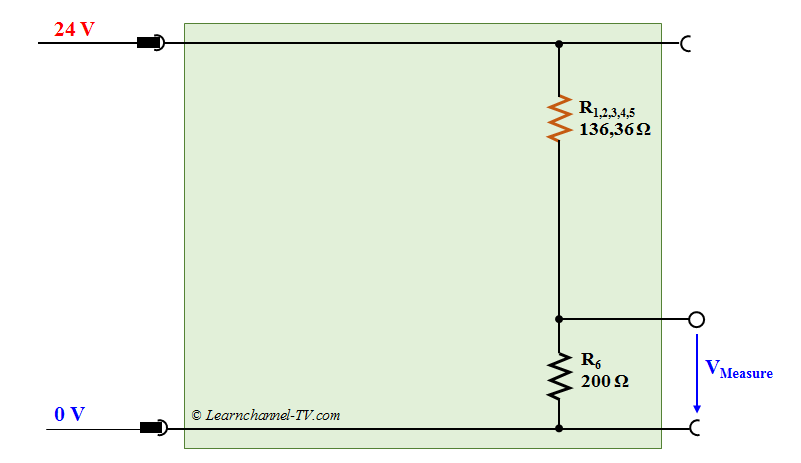# Resistors in a Mixed Connection

### Resistors in a Mixed Connection

Look at which resistors are in parallel or in series and summarize them. Work from "inside" to "outside". Here is an example: The expected measurement voltage is to be calculated. Therefor, you have to determine the total resistance:Resistors in Mixed Connection 1

Step 1: R1, R2 and R3 are parallel and can be combined to form a substitute response R1,2,3.

R1,2,3 = R1/3 = 300 Ω / 3 = 100 ΩResistors in Mixed Connection 2

Step 2: You can now see that R1,2,3 and R4 are in series. The equivalent resistance R1,2,3,4 can easily be determined by addition:

R1,2,3,4 = R1,2,3 + R4 = 100 Ω + 150 Ω = 250 Ω

This results in the following equivalent circuit:Resistors in Mixed Connection 3

Step 3: Now we dissolve the parallel connection of R1,2,3,4 and R5:

R1,2,3,4,5   =   R1,2,3,4  *   R5     =  250 Ω  *  300 Ω   =  136,36 Ω
.                    R1,2,3,4  +  R5         250 Ω  +  300 ΩResistors in Mixed Connection 4

Step 4: What remains is a simple series connection. Calculating the total current which flows through R6 is now a simple exercise:

Rt = R1,2,3,4,5 + R6  = 136,36 Ω  + 200 Ω = 336,36 Ω

It  =  IR6   =   U   =       24 V         = 71,35 mA
.                    Rt       336,36 Ω

=> VMeasure = UR6 * It    = 200 Ω  *  71,35 mA = 14,27 V